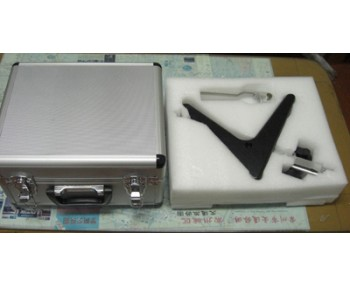A professional manufacture and sales of various electronic balances, moisture analyzers, viscometers and laboratory instruments.
weighinginstru@gmail.com

# How to Choose a Viscometer?

2020/07/07

Fluids can be divided into Newtonian and non-Newtonian according to major categories. Non-Newtonian fluids are further divided into shear rate-dependent and time-dependent types. Shear rate dependent type refers to the flow behavior of fluid changes with the change of shear rate, including pseudo-plastic type, dilatant type and plastic type. The time-dependent type refers to the flow characteristics of fluid changing with time at a certain shear rate, including thixotropic type and shock-coagulation type.

Scientist Newton found that the ratio of shear stress τ to shear rate D when some fluids flow is constant, that is: η=τ/D. Both water and oil are fluids that follow the above rules. This formula is Newton's law of viscosity. where η is the viscosity of the fluid. Viscosity is a measure of internal friction or resistance when the fluid flows. The unit of η is mPa·s or Pa·s (Pascal seconds). Newtonian fluid is a mixed single fluid without particles. The fluid whose viscosity does not change with the change of shear stress is called Newtonian fluid, and the fluid whose viscosity changes with the change of shear stress is called non-Newtonian fluid.

Therefore, when using a digital rotational viscometer, the viscosity value for Newtonian fluid is certain. As long as the viscosity value is within the current measurement allowable range (torque is 20%-90%), choose a different rotor and The speed can get the same result. Our digital viscometer basically stabilizes the reading after the rotor (such as L1-L4 standard rotor) rotates 5-6 revolutions. For example, the selected speed is 30 revolutions per minute, that is, one revolution in 2 seconds, about 10 -12 seconds to read. The measurement range marked by the viscosity meter refers to the viscosity of Newtonian fluid.Viscosity Meter

But for non-Newtonian fluids, the viscosity value is not fixed, because the shear rate provided by different rotors and rotational speeds is different, so the specific phenomena that may be encountered are:

1. After selecting a certain rotor and a certain speed, the viscosity value changes rapidly from large to small, and gradually stabilizes (the stability refers to the measured torque value changes each time between 0.1%-0.2%), due to our figures The viscosity of the viscometer is very high, so it is often seen that the viscosity value continues to decrease after a long time of measurement, and the decrease is getting smaller and smaller, which is in line with the characteristics of non-Newtonian fluids.

2. After selecting a certain rotor and a certain speed, the viscosity value increases rapidly from small to small, and gradually stabilizes.

3. The viscosity value is different after selecting the same rotor and different speeds.

4. Viscosity values are different after selecting different rotors and the same speed. Therefore, the elements that need to be determined for the measurement of non-Newtonian fluids are the rotor, speed, and time. Only when these elements are fixed can several comparisons be made. Generally, these elements are determined by users according to their own purposes and requirements through experiments. Usually the choice of rotor and speed should meet the measured viscosity value within the current measurement allowable range (torque is 20%-90%), the time is determined from the start to the measured torque value change range is 0.1%- 0.2% end. For example, for a certain sample, choose the No. L4 rotor, 30 rpm, and it will be stable in 5 minutes (the torque value varies from 0.1% to 0.2%). At this time, the viscosity is 10000mPa·S and the torque is 50%. Then, if you need to compare with similar samples in the future, under the same measurement conditions, that is, the L4 rotor, 30 revolutions per minute, and 5 minutes of reading.

In addition, the influence of temperature on the viscosity is also very large, the temperature control of similar samples must be better.